Home

# THE LATTICE OF VARIETIES OF STRICT LEFT RESTRICTION SEMIGROUPS

## Abstract

Left restriction semigroups are the unary semigroups that abstractly characterize semigroups of partial maps on a set, where the unary operation associates to a map the identity element on its domain. This paper is the sequel to two recent papers by the author, melding the results of the first, on membership in the variety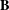$\mathbf{B}$ of left restriction semigroups generated by Brandt semigroups and monoids, with the connection established in the second between subvarieties of the variety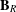$\mathbf{B}_{R}$ of two-sided restriction semigroups similarly generated and varieties of categories, in the sense of Tilson. We show that the respective lattices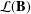${\mathcal{L}}(\mathbf{B})$ and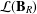${\mathcal{L}}(\mathbf{B}_{R})$ of subvarieties are almost isomorphic, in a very specific sense. With the exception of the members of the interval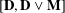$[\mathbf{D},\mathbf{D}\vee \mathbf{M}]$ , every subvariety of$\mathbf{B}$ is induced from a member of$\mathbf{B}_{R}$ and vice versa. Here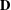$\mathbf{D}$ is generated by the three-element left restriction semigroup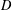$D$ and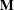$\mathbf{M}$ is the variety of monoids. The analogues hold for pseudovarieties.

## References

Hide All
MathJax
MathJax is a JavaScript display engine for mathematics. For more information see http://www.mathjax.org.

# THE LATTICE OF VARIETIES OF STRICT LEFT RESTRICTION SEMIGROUPS

## Metrics

### Full text viewsFull text views reflects the number of PDF downloads, PDFs sent to Google Drive, Dropbox and Kindle and HTML full text views.

Total number of HTML views: 0
Total number of PDF views: 0 *Loading metrics...

### Abstract viewsAbstract views reflect the number of visits to the article landing page.

Total abstract views: 0 *Loading metrics...

* Views captured on Cambridge Core between <date>. This data will be updated every 24 hours.

Usage data cannot currently be displayed